Courses

# Plane Table Surveying & Calculation Of Area And Volume - 2

## 10 Questions MCQ Test Topicwise Question Bank for GATE Civil Engineering | Plane Table Surveying & Calculation Of Area And Volume - 2

Description
This mock test of Plane Table Surveying & Calculation Of Area And Volume - 2 for Civil Engineering (CE) helps you for every Civil Engineering (CE) entrance exam. This contains 10 Multiple Choice Questions for Civil Engineering (CE) Plane Table Surveying & Calculation Of Area And Volume - 2 (mcq) to study with solutions a complete question bank. The solved questions answers in this Plane Table Surveying & Calculation Of Area And Volume - 2 quiz give you a good mix of easy questions and tough questions. Civil Engineering (CE) students definitely take this Plane Table Surveying & Calculation Of Area And Volume - 2 exercise for a better result in the exam. You can find other Plane Table Surveying & Calculation Of Area And Volume - 2 extra questions, long questions & short questions for Civil Engineering (CE) on EduRev as well by searching above.
QUESTION: 1

Solution:
QUESTION: 2

Solution:
QUESTION: 3

### The quick and most accurate method to solve three point problem in resection type of plane tabling is

Solution:
QUESTION: 4

The ‘fix’ of a plane table with three known points is good if the instrument station lies

Solution:
QUESTION: 5

Consider the following steps
1. Using Lehmann's rule, an improved position of station point is obtained
2. Procedure is repeated till the triangle of error is reduced to a point
3. Triangle of error is obtained
4. The plane table is levelled over the station point
5. Three resection tines are drawn from three well defined station points

The correct sequence of "Lehman’s Procedure" for solving the three point problem is

Solution:
QUESTION: 6

Consider the following statements regarding Plane Table surveying
1. It is less accurate than chain surveying
2. It is not necessary to do accurate centering of plane table for small scale surveys
3. Compass rule may be made use of for adjusting the plane table traverse
4. From the instrument station, resectors are drawn to plot the position of objects in the field

Which of these statements is/are correct?

Solution:
QUESTION: 7

Consider the following statements pertaining to plane table survey
1. Two-point problem is solved by mechanical method.
2. Three-point problem is solved by Bessel’s method
3. In two-point problem, auxiliary station is required

Which of these statements is/are correct?

Solution:
QUESTION: 8

Which one of the following instruments is used in plane table surveying for the measurement of horizontal and vertical distances directly?

Solution:
QUESTION: 9

In the given formula formats, L is the length of a base line split into ‘n’ equal segments each of length ‘d’. O1, O2 .......On+1 are the ordinates at the sequential ends of the segments and M1, M2....Mn are the mid-ordinates of successive segments. Which of the following pairs of rules and the formulae for computation of the area standing on the base line are correctly matched?
1. Mid - ordinate Rule ...............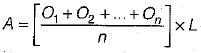2. Average - ordinate Rule ......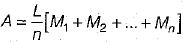3. Trapezoidal Rule........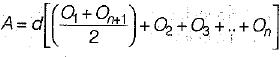4. Simpsons’ rule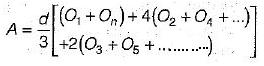Select the correct answer using the codes given below

Solution: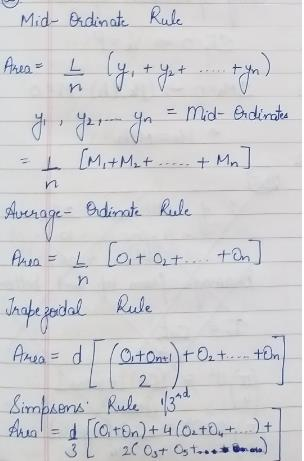QUESTION: 10

Which one of the following methods estimates best the area of an irregular and curved boundary?

Solution:

Mid-ordinate and Average ordinate methods are used with the assumption that the boundaries between the extremities of the ordinates are straight lines.
Trapezoidal method is based on the assumption that the figures are trapezoids. The method is more accurate than the above two methods.
Simpson’s method assumes that the short lengths of the boundaries between the ordinates are parabolic arcs. This method is more useful when the boundary line departs considerably from the straight line. The results obtained by use of Simpson’s method in all cases are more accurate.